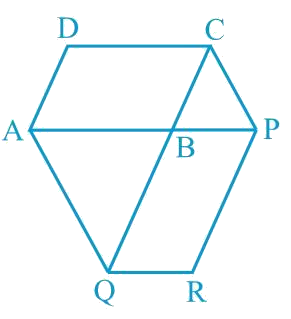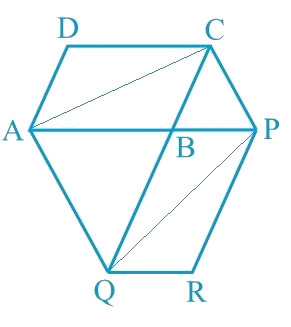# Ex.9.3 Q9 Areas of Parallelograms and Triangles Solution - NCERT Maths Class 9

## Question

The side $$AB$$ of a parallelogram $$ABCD$$ is produced to any point $$P$$. $$A$$ line through $$A$$ and parallel to $$CP$$ meets $$CB$$ produced at $$Q$$ and then parallelogram $$PBQR$$ is completed (see the following figure). Show that $$ar (ABCD) = ar (PBQR)$$.

[Hint: Join $$AC$$ and $$PQ$$. Now compare area $$(ACQ)$$ and area $$(APQ)$$]Video Solution
Areas Of Parallelograms And Triangles
Ex 9.3 | Question 9

## Text Solution

What is known?

The side AB of a parallelogram $$ABCD$$ is produced to any point $$p$$. A line through A and parallel to $$CP$$ meets $$CB$$ produced at $$Q$$ and then parallelogram $$PBQR$$ is completed.

What is unknown?

How we can show that $$ar (ABCD) = ar (PBQR).$$

Reasoning:

First of all, we can join $$AC$$ and $$PQ$$. Now we can use theorem for triangles $$ACQ$$ and $$AQP$$ if two triangles are on same base and between same pair of parallel lines then both will have equal area. Now we can subtract common area of triangle $$ABQ$$ from both sides. Now we can compare the result with half of which we have to show.

Steps:Let us join $$AC$$ and $$PQ$$.

$$\Delta ACQ$$ and $$\Delta AQP$$ are on the same base $$AQ$$ and between the same parallels $$AQ$$ and $$CP$$.

According to Theorem 9.2: Two triangles on the same base (or equal bases) and between the same parallels are equal in area.

\begin{align} \text {ar}(\Delta {ACQ})&=\text {ar}(\Delta {APQ})\end{align}

$\,\left[ \begin{array}{l}{\rm{ar}}(\Delta {{ACQ}})\, -\\{\rm{ar}}(\Delta {{ABQ}})\end{array} \right]\!\!=\!\!\left[ \begin{array}{l}{\rm{ar}}(\Delta {{APQ}})\, - \\{{ar}}(\Delta {{ABQ}})\end{array} \right]$

Subtracting Area $$(\Delta {ABQ})$$ on both the sides.

\begin{align} (\Delta {ABC})&=\text {ar}(\Delta {QBP}) ...(1)\end{align}

Since  and  are diagonals of parallelograms  and  respectively,

\begin{align}\text {ar}(\Delta {ABC})&=\frac{1}{2} \text {ar}({ABCD})...(2) \\ \text {ar}(\Delta {QBP})&=\frac{1}{2} {ar(PBQR) } ...(3)\end{align}

From Equations (), (), and (), we obtain

\begin{align}\frac{1}{2} \text {ar}({ABCD})&=\frac{1}{2} \text {ar}(PBQR) \\ \text {ar}({ABCD})&=\text {ar}({PBQR})\end{align}

Video Solution
Areas Of Parallelograms And Triangles
Ex 9.3 | Question 9
Learn from the best math teachers and top your exams

• Live one on one classroom and doubt clearing
• Practice worksheets in and after class for conceptual clarity
• Personalized curriculum to keep up with school